# IF Tank Design for the MAX2360

### 要約

This application note presents three voltage-controlled oscillator (VCO) designs for popular IF frequencies of 130MHz, 165MHz, and 380MHz. These designs reduce the number of iterations required for optimized results. Analysis can be accomplished with a simple spreadsheet program.

### Introduction

This application note presents three voltage-controlled oscillator (VCO) designs for popular IF frequencies of 130MHz, 165MHz, and 380MHz. These designs reduce the number of iterations required for optimized results. Analysis can be accomplished with a simple spreadsheet program.

### VCO Design

Figure 2 shows the differential tank circuit used for the MAX2360 IF VCO.For analysis purposes, the tank circuit must be reduced to an equivalent simplified model. Figure 1 depicts the basic VCO model. The frequency of oscillation can be characterized by EQN1:fosc = frequency of oscillation
L = inductance of the coil in the tank circuit
Cint = internal capacitance of the MAX2360 tank port
Ct = total equivalent capacitance of the tank circuit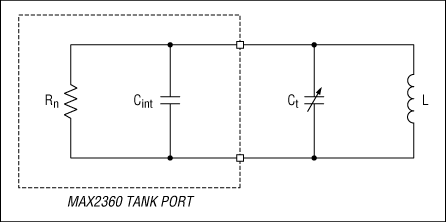Rn = equivalent negative resistance of the MAX2360 tank port
Cint = internal capacitance of the MAX2360 tank port
Ct = total equivalent capacitance of the tank circuit
L = inductance of the coil in the tank circuitInductor L resonates with the total equivalent capacitance of the tank and the internal capacitance of the oscillator (Ct + Cint) (see Figure 1). Ccoup provides DC block and couples the variable capacitance of the varactor diodes to the tank circuit. Ccent is used to center the tank's oscillationfrequency to a nominal value. It is not required but adds a degree of freedom by allowing you to fine-tune resonance between inductor values. Resistors (R) provide reverse-bias voltage to the varactor diodes via the tune voltage line (Vtune). Their value should be chosen large enough so as not to affect loaded tank Q but small enough so that 4kTBR noise is negligible. The resistors' noise voltage gets modulated by Kvco, producing phase noise. Capacitance CV is the variable tuning component in the tank. The capacitance of the varactor diode (CV) is a function of reverse-bias voltage (see Appendix A for the varactor model). Vtune is the tuning voltage from a phase-locked loop (PLL).

Figure 3 shows the lumped Cstray VCO model. Parasitic capacitance and inductance plague every RF circuit. In order to predict the frequency of oscillation, the parasitic elements must be taken into account. The circuit in Figure 3 lumps the parasitic elements in one capacitor called Cstray. The frequency of oscillation can be characterized by EQN2:L = inductance of the coil in the tank circuit
Cint = internal capacitance of the MAX2360 tank port
Ccent = tank capacitor used to center oscillation frequency
Cstray = lumped stray capacitance
Ccoup = tank capacitor used to couple the varactor to the tank
CV = net variable capacitance of the varactor diode (including series inductance)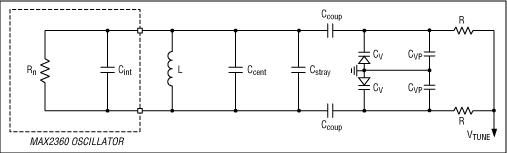Figure 4 depicts the detailed VCO model. It takes into account the capacitance of the pads but does not include the effects of series inductance for simplicity. Cstray is defined as: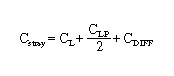CL = capacitance of the inductor
CLP = capacitance of inductor pads
CDIFF = capacitance due to parallel tracesRn = equivalent negative resistance of the MAX2360 tank port
Cint = internal capacitance of the MAX2360 tank port
LT = inductance of series trace to the inductor tank circuit
CDIFF = capacitance due to parallel traces
L = inductance of the coil in the tank circuit
CL = capacitance of the inductor
CLP = capacitance of inductor pads
Ccent = tank capacitor used to center oscillation frequency
Ccoup = tank capacitor used to couple the varactor to the tank
Cvar = variable capacitance of the varactor diode
LS = series inductance of the varactor
R = resistance of varactor reverse-bias resistors

To simplify analysis, inductance LT is ignored in this design. The effects of LT are more pronounced at higher frequencies. To mathematically model the shift in frequency due to LT with the spreadsheets that follow, the value of CDIFF can be increased appropriately. Minimize inductance LT to prevent undesired series resonance. This can be accomplished by making the traces short.

### Tuning Gain

Tuning gain (Kvco) must be minimized for best closed-loop phase noise. Resistors in the loop filter as well as the resistors "R" (Figure 2) will produce broadband noise. Broadband thermal noise (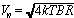) will modulate the VCO by Kvco, which is measured in MHz/V. There are two ways to minimize Kvco. One is to minimize the frequency range over which the VCO must tune. The second way is to maximize the tuning voltage available. To minimize the frequency range over which the VCO must tune, tight tolerance components must be used, as will be shown. To maximize tuning voltage, a charge pump with a large compliance range is needed. This is usually accomplished by using a larger Vcc. The compliance range for the MAX2360 is 0.5V to Vcc-0.5V. In battery-powered applications, the compliance range is usually fixed by the battery voltage or regulator.

### Basic Concept for Trimless Design

VCO design manufacturability with real-world components will require an error budget analysis. In order to design a VCO to oscillate at a fixed frequency (fosc), the tolerance of components must be taken into consideration. Tuning gain (Kvco) must be designed into the VCO to account for these component tolerances. The tighter the component tolerance, the smaller the tuning gain and the lower the closed-loop phase noise. For the worst-case error budget design, we will look at three VCO models:

1. Maximum-value components (EQN5)
2. Nominal tank, all components perfect (EQN2)
3. Minimum-value components (EQN4)

All three VCO models must cover the desired nominal frequency. Figure 5 shows how the three designs must converge to provide a manufacturable design solution. Observations of EQN1 and Figure 5 reveal that minimum-value components shift the oscillation frequency higher, and maximum-value components shift the oscillation frequency lower.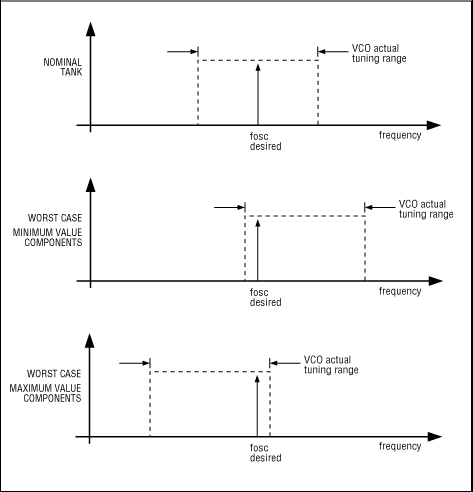Minimum tuning range must be used in order to design a tank with the best closed-loop phase noise. Therefore, the nominal tank should be designed to cover the center frequency with overlap to take into account device tolerance. The worst-case high-tune tank and worst-case low-tune tank should tune just to the edge of the desired oscillation frequency. EQN2 can be modified by component tolerance to produce a worst-case high-tune tank EQN4 and worst-case low-tune tank EQN5.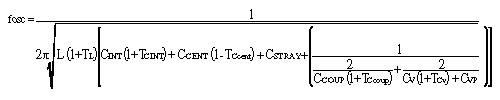TL = % tolerance of inductor (L)
TCINT = % tolerance of capacitor (CINT)
TCCENT = % tolerance of capacitor (CCENT)
TCCOUP = % tolerance of capacitor (CCOUP)
TCV = % tolerance of varactor capacitance (CV)
EQN4 and EQN5 assume that the strays do not have a tolerance.

### General Design Procedure

#### Step 1

Estimate or measure pad capacitance and other strays. The stray capacitance on the MAX2360 Rev A EV Kit has been measured with a Boonton Model 72BD capacitance meter. CLP = 0.981pF, CVP = 0.78pF, CDIFF = 0.118pF.

#### Step 2

Determine the value for capacitance Cint. This can be found in the MAX2360/MAX2362/MAX2364 Data Sheet on page 5. The typical operating characteristic TANK 1/S11 vs. FREQUENCY shows the equivalent parallel RC values for several popular LO frequencies. Keep in mind that the LO frequency is twice the IF frequency.

#### Example:

For an IF frequency of 130MHz, the LO operates at 260MHz. From the 1/s11 chart, Rn = -1.66kΩ and Cint = 0.31pF.

#### Step 3

Choose an inductor. A good starting point is using the geometric mean. This is an iterative process.This equation assumes L in (nH) and C in (pF) (1x10-9 x 1x10-12 = 1x10-21). L = 19.3nH for a fosc = 260.76MHz. This implies a total tank capacitance C = 19.3pF. An appropriate initial choice for an inductor would be 18nH Coilcraft 0603CS-18NXGBC 2% tolerance.

When choosing an inductor with finite step sizes, the following formula EQN6.1 is useful. The total product LC should be constant for a fixed oscillation frequency fosc.LC = 372.5 for a fosc = 260.76MHz. The trial-and-error process with the spreadsheet in Table1 yielded an inductor value of 39nH 5% with a total tank capacitance of 9.48pF. The LC product for the tank in Figure 6 is 369.72, which is close enough to the desired LC product of 372.5. One can see this is a useful relationship to have on hand. For best phase noise, choose a high Q inductor like the Coilcraft 0603CS series. Alternatively, a microstrip inductor can be used if the tolerance and Q can be controlled reasonably.#### Step 4

Determine the PLL compliance range. This is the range the VCO tuning voltage (Vtune) is designed to work over. For the MAX2360, the compliance range is 0.5V to VCC-0.5V. For a VCC = 2.7V, this would set the compliance range to 0.5 to 2.2V. The charge-pump output sets this limit. The voltage swing on the tank is 1VP-P centered at 1.6VDC. Even with large values for Ccoup, the varactor diodes are not forward-biased. This is a condition to be avoided, as the diode rectifies the AC signal on the tank pins, producing undesirable spurious response and loss of lock in a closed-loop PLL.

#### Step 5

Choose a varactor. Look for a varactor with good tolerance over your specified compliance range. Keep the series resistance small. For a figure of merit, check that the self-resonant frequency of the varactor is above the desired operating point. Look at the CV(2.5V)/CV(0.5V) ratio at your voltage compliance range. If the coupling capacitors Ccoup were chosen large, then the maximum tuning range can be calculated using EQN2. Smaller values of capacitor Ccoup reduce this effective frequency tuning range. When choosing a varactor, it should have a tolerance specified at your given compliance-range mid and end points. Select a hyperabrupt varactor such as the Alpha SMV1763-079 for the linear tuning range. Take the value for total tank capacitance, and use that for Cjo of the varactor. Remember that Ccoup reduces the net capacitance coupled to the tank.

#### Step 6

Pick a value for Ccoup. Large values of Ccoup increase the tuning range by coupling more of the varactor in the tank at the expense of decreasing tank loaded Q. Smaller values of Ccoup increase the effective Q of the coupled varactor and loaded Q of the tank at the expense of reducing the tuning range. Typically this value is chosen as small as possible, while still getting the desired tuning range. Another benefit of choosing a small value for Ccoup is that it reduces the voltage swing across the varactor diode. This helps thwart forward-biasing the varactor.

#### Step 7

Pick a value for Ccent, which is usually around 2pF or greater for tolerance purposes. Use Ccent to center up the VCO's frequency.

### MAX2360VCO Tank Designs for IF Frequencies of 130.38MHz, 165MHz, and 380MHz

The following spreadsheets show designs for several popular IF frequencies for the MAX2360. Keep in mind that the LO oscillates at twice the desired IF frequency.

 Light grey indicates calculated values

 Darker grey indicates user input
 MAX2360 Tank Design and Tuning Range for 130.38MHz IF Frequency Total Tank Capacitance vs. V tune V tune Total C Ct (Nominal) Ct (Low) Ct (High) 0.5V Ct high 10.9296pF 10.1242pF 11.6870pF 1.375V Ct mid 9.4815pF 8.4068pF 10.4077pF 2.2V Ct low 8.0426pF 6.9014pF 9.0135pF
 Tank Components Tolerance C coup 18pF 0.9pF 5% C cent 2.7pF 0.1pF 4% C stray 0.69pF L 39nH 5.00% C int 0.31pF 10.00%
 Parasitics and Pads (C stray) Due to Q C L 0.08pF Ind. pad C Lp 0.981pF Due to || C diff 0.118pF Var. pad C vp 0.78pF
 Varactor Specs Alpha SMV1255-003 Cjo 82pF Varactor Tolerance Vj 17V 0.5V 19.00% M 14 1.5V 29.00% Cp 0pF 2.5V 35.00% Rs 1Ω Reactance Ls 1.7nH X Ls 2.79 Freq 260.76MHz
 Nominal Varactor X c Net Cap Cv high 54.64697pF -11.16897 72.80216pF Cv mid 27.60043pF -22.11379 31.57772pF Cv low 14.92387pF -40.89758 16.01453pF
 Negative Tol Varactor (Low Capacitance) Cv high 44.26404pF -13.78885 55.46841pF Cv mid 19.59631pF -31.14619 21.52083pF Cv low 9.700518pF -62.91935 10.14983pF
 Positive Tol Varactor (High Capacitance) Cv high 65.02989pF -9.385688 92.47168pF Cv mid 35.60456pF -17.14248 42.51182pF Cv low 20.14723pF -30.2945 22.18712pF
 Nominal LO (Nom) Range Low Tol IF (High) Range Nominal IF (Nom) Range High Tol IF(Low) Range F low 243.77MHz 129.93MHz 121.89MHz 115.03MHz F mid 261.73MHz 142.59MHz 130.86MHz 121.90MHz F high 284.18MHz 157.37MHz 142.09MHz 130.98MHz BW 40.40MHz 27.44MHz 20.20MHz 15.95MHz % BW 15.44% 19.24% 15.44% 13.09%
 Nominal IF Frequency 130.38MHz
 Design Constraints Condition for bold number IF Delta 0.45 -0.48 0.60 Test pass pass pass Raise or lower cent freq by -0.48 MHz Inc or dec BW -1.05 MHz Cent adj for min BW 130.46 MHz
 K vco 23.77MHz/VLight grey indicates calculated values

 Darker grey indicates user input
 MAX2360 Tank Design and Tuning Range for 165MHz IF Frequency Total Tank Capacitance vs. V tune V tune Total C Ct (Nominal) Ct (Low) Ct (High) 0.5V Ct high 10.0836pF 9.2206pF 10.8998pF 1.375V Ct mid 8.5232pF 7.3878pF 9.5095pF 2.2V Ct low 7.0001pF 5.8130pF 8.0193pF
 Tank Components Tolerance C coup 18pF 0.9pF 5% C cent 1.6pF 0.1pF 6% C stray 0.62pF L 27nH 5.00% C int 0.34pF 10.00%
 Parasitics and Pads (C stray) Due to Q C L 0.011pF Ind. pad C Lp 0.981pF Due to || C diff 0.118pF Var. pad 18C vppF 0.78pF
 Varactor Specs Alpha SMV1255-003 Cjo 82pF Varactor Tolerance Vj 17V 0.5V 19.00% M 14 1.5V 29.00% Cp 0pF 2.5V 35.00% Rs 1ohm Reactance Ls 1.7nH X Ls 3.52 Freq 330.00MHz
 Nominal Varactor X c Net Cap Cv high 54.646968pF -8.8255163 90.986533pF Cv mid 27.600432pF -17.473919 34.574946pF Cv low 14.923873pF -32.316524 16.750953pF
 Negative Tol Varactor (Low Capacitance) Cv high 44.264044pF -10.895699 65.431921pF Cv mid 19.596307pF -24.611153 22.872103pF Cv low 9.7005176pF -49.717729 10.440741pF
 Positive Tol Varactor (High Capacitance) Cv high 65.029892pF -7.4164003 123.93257pF Cv mid 35.604558pF -13.545673 48.128632pF Cv low 20.147229pF -23.938166 23.626152pF
 Nominal LO (Nom) Range Low Tol IF (High) Range Nominal IF (Nom) Range High Tol IF (Low) Range F low 305.02MHz 163.63MHz 152.51MHz 143.15MHz F mid 331.77MHz 182.81MHz 165.88MHz 153.26MHz F high 366.09MHz 206.08MHz 183.04MHz 166.90MHz BW 61.07MHz 42.45MHz 30.53MHz 23.74MHz % BW 18.41% 23.22% 18.41% 15.49%
 Nominal IF Frequency 165MHz
 Design Constraints Condition for bold number < IF = IF > IF Delta 1.37 -0.88 1.90 Test pass pass pass Raise or lower cent freq by -0.88 MHz Inc or dec BW -3.26 MHz Cent adj for min BW 165.26 MHz
 K vco 35.92MHz/V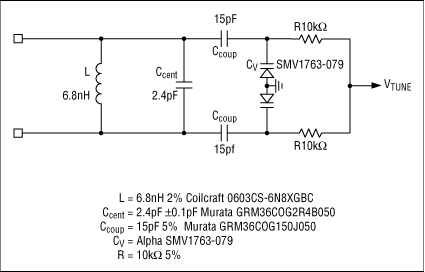Light grey indicates calculated values

 Darker grey indicates user input
 MAX2360 Tank Design and Tuning Range for 380MHz IF Frequency Total Tank Capacitance vs. V tune V tune Total C Ct (Nominal) Ct (Low) Ct (High) 0.5V Ct high 6.9389pF 6.6119pF 7.2679pF 1.35V Ct mid 6.2439pF 5.9440pF 6.5449pF 2.2V Ct low 5.7813pF 5.5040pF 6.0593pF
 Tank Components Tolerance C coup 15pF 0.8pF 5% C cent 2.4pF 0.1pF 4% C stray 1.42pF L 6.8nH 2.00% C int 0.43pF 12310.00
 Parasitics and Pads (C stray) Due to Q C L 0.08pF Ind. pad C Lp 0.981pF Due to || C diff 0.85pF Var. pad C vp 0.78pF
 Varactor Specs Alpha SMV1255-003 Cjo 8.2pF Varactor Tolerance Vj 15V 0.5V 7.50% M 9.5 1.5V 9.50% Cp 0.67pF 2.5V 11.50% Rs 0.5Ω Reactance Ls 0.8nH X Ls 3.82 Freq 760.00MHz
 Nominal Varactor X c Net Cap Cv high 6.67523pF -31.37186 7.600784pF Cv mid 4.286281pF -48.8569 4.649858pF Cv low 2.904398pF -72.10251 3.06689pF
 Negative Tol Varactor (Low Capacitance) Cv high 6.174588pF -33.91552 6.958364pF Cv mid 3.879084pF -53.98553 4.174483pF Cv low 2.570392pF -81.47176 2.696846pF
 Positive Tol Varactor (High Capacitance) Cv high 7.175873pF -29.18313 8.256705pF Cv mid 4.693477pF -44.61818 5.132957pF Cv low 3.238404pF -64.66593 3.441726pF
 Nominal LO (Nom) Range Low Tol IF (High) Range Nominal IF (Nom) Range High Tol IF (Low) Range F low 732.69MHz 379.11MHz 366.35MHz 354.43MHz F mid 772.40MHz 399.84MHz 386.20MHz 373.50MHz F high 802.70MHz 415.51MHz 401.35MHz 388.17MHz BW 70.00MHz 36.41MHz 35.00MHz 33.74MHz % BW 9.06% 9.11% 9.06% 9.03%
 Nominal IF Frequency 380MHz
 Design Constraints Condition for bold number < IF = IF > IF Delta 0.89 -6.20 8.17 Test pass pass pass Raise or lower cent freq by -6.20 MHz Inc or dec BW -9.07 MHz Cent adj for min BW 383.64 MHz
 K vco 41.18MHz/V

### Appendix A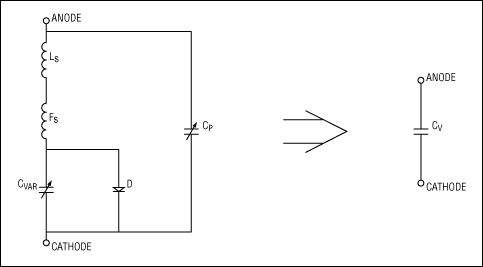Alpha Application Note AN1004 has additional information on varactor models. Varactor capacitance is defined in EQN7.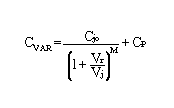Alpha SMV1255-003 Alpha SMV1763-079 Cjo = 82 pF Cjo = 8.2 pF Vj =17 V Vj =15 V M = 14 M = 9.5 Cp = 0 Cp = 0.67 Rs = 1Ω Rs = 0.5Ω Ls = 1.7 nH Ls = 0.8 nH

The series inductance of the varactor is taken into account by backing out the inductive reactance and calculating a new effective capacitance CV.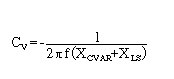### 参考資料

1. Chris O'Connor, Develop Trimless Voltage-Controlled Oscillators, Microwaves and RF, July 1999.
2. Wes Hayward, Radio Frequency Design, Chapter 7.
3. Krauss, Bostian, Raab, Solid State Radio Engineering, Chapters 2, 3, 5.
4. Alpha Industries Application Note AN1004.
5. Coilcraft, RF Inductors Catalog, March 1998, p.131.
6. Maxim, MAX2360/MAX2362/MAX2364 Data Sheet Rev 0.
7. Maxim, MAX2360 Evaluation Kit Data Sheet Rev 0.